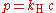xGas lawsEncyclopedia
The early gas laws were developed at the end of the 18th century, when scientists began to realize that relationships between the pressure, volume and temperature of a sample of gas could be obtained which would hold for all gases. Gases behave in a similar way over a wide variety of conditions because to a good approximation they all have molecules which are widely spaced, and nowadays the equation of state for an ideal gas is derived from kinetic theory
Kinetic theory
The kinetic theory of gases describes a gas as a large number of small particles , all of which are in constant, random motion. The rapidly moving particles constantly collide with each other and with the walls of the container...

. The earlier gas laws are now considered as special cases of the ideal gas equation, with one or more of the variables held constant.

## Boyle's law

Boyle's law shows that, at constant temperature, the product of an ideal gas's
Ideal gas
An ideal gas is a theoretical gas composed of a set of randomly-moving, non-interacting point particles. The ideal gas concept is useful because it obeys the ideal gas law, a simplified equation of state, and is amenable to analysis under statistical mechanics.At normal conditions such as...

pressure and volume is always constant. It was published in 1662. It can be determined experimentally using a pressure gauge and a variable volume container. It can also be found through the use of logic; if a container, with a fixed number of molecules inside, is reduced in volume, more molecules will hit the sides of the container per unit time, causing a greater pressure.

As a mathematical equation, Boyle's law is: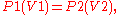v =k/p where k is the constant of proportionality and its value is 1

Where P is the pressure (Pa), V the volume (m3) of a gas, and k1 (measured in joule
Joule
The joule ; symbol J) is a derived unit of energy or work in the International System of Units. It is equal to the energy expended in applying a force of one newton through a distance of one metre , or in passing an electric current of one ampere through a resistance of one ohm for one second...

s) is the constant from this equation—it is not the same as the constants from the other equations below.

## Charles's law

Charles's Law, or the law of volumes, was found in 1678. It says that, for an ideal gas at constant pressure, the volume is directly proportional to the absolute temperature (in kelvin
Kelvin
The kelvin is a unit of measurement for temperature. It is one of the seven base units in the International System of Units and is assigned the unit symbol K. The Kelvin scale is an absolute, thermodynamic temperature scale using as its null point absolute zero, the temperature at which all...

s).

This can be found using the kinetic theory of gases or a heated or cooled [not frozen <0] container with a variable volume (such as a conical flask with a balloon).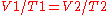Where T is the absolute temperature of the gas (in kelvins) and k2 (in m3·K−1) is the constant produced.

## Gay-Lussac's law

Gay-Lussac's law, or the pressure law, was found by Joseph Louis Gay-Lussac
Joseph Louis Gay-Lussac
- External links :* from the American Chemical Society* from the Encyclopædia Britannica, 10th Edition * , Paris...

in 1809. It states that the pressure exerted on a container's sides by an ideal gas is proportional to the absolute temperature of the gas. This follows from the kinetic theory—by increasing the temperature of the gas, the molecules' speeds increase meaning an increased number of collisions with the container walls.

As a mathematical formula, this is: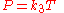Avogadro's law states that the volume occupied by an ideal gas is proportional to the number of moles
Mole (unit)
The mole is a unit of measurement used in chemistry to express amounts of a chemical substance, defined as an amount of a substance that contains as many elementary entities as there are atoms in 12 grams of pure carbon-12 , the isotope of carbon with atomic weight 12. This corresponds to a value...

(or molecules) present in the container. This gives rise to the molar volume
Molar volume
The molar volume, symbol Vm, is the volume occupied by one mole of a substance at a given temperature and pressure. It is equal to the molar mass divided by the mass density...

of a gas, which at STP
Standard conditions for temperature and pressure
Standard condition for temperature and pressure are standard sets of conditions for experimental measurements established to allow comparisons to be made between different sets of data...

is 22.4 dm3 (or litres).

equation P1 / T1 = P2 / T2

Where n is equal to the number of moles of gas (the number of molecules divided by Avogadro's Number
In chemistry and physics, the Avogadro constant is defined as the ratio of the number of constituent particles N in a sample to the amount of substance n through the relationship NA = N/n. Thus, it is the proportionality factor that relates the molar mass of an entity, i.e...

).

## Combined and ideal gas laws

The combined gas law
Combined gas law
The combined gas law is a gas law which combines Charles's law, Boyle's law, and Gay-Lussac's law. These laws each relate one thermodynamic variable to another mathematically while holding everything else constant. Charles's law states that volume and temperature are directly proportional to each...

or general gas equation is formed by the combination of the three laws, and shows the relationship between the pressure, volume, and temperature for a fixed mass of gas: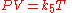Avogadro's law is a gas law named after Amedeo Avogadro who, in 1811, hypothesized that two given samples of an ideal gas, at the same temperature, pressure and volume, contain the same number of molecules...

, the combined gas law
Combined gas law
The combined gas law is a gas law which combines Charles's law, Boyle's law, and Gay-Lussac's law. These laws each relate one thermodynamic variable to another mathematically while holding everything else constant. Charles's law states that volume and temperature are directly proportional to each...

develops into the ideal gas law
Ideal gas law
The ideal gas law is the equation of state of a hypothetical ideal gas. It is a good approximation to the behavior of many gases under many conditions, although it has several limitations. It was first stated by Émile Clapeyron in 1834 as a combination of Boyle's law and Charles's law...

: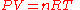Where the constant, now named R, is the gas constant
Gas constant
The gas constant is a physical constant which is featured in many fundamental equations in the physical sciences, such as the ideal gas law and the Nernst equation. It is equivalent to the Boltzmann constant, but expressed in units of energy The gas constant (also known as the molar, universal,...

with a value of .08206 (atm∙L)/(mol∙K)
An equivalent formulation of this law is: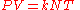where
k is the Boltzmann constant (1.381×10−23 J·K−1 in SI units)
N is the number of molecules.

These equations are exact only for an ideal gas
Ideal gas
An ideal gas is a theoretical gas composed of a set of randomly-moving, non-interacting point particles. The ideal gas concept is useful because it obeys the ideal gas law, a simplified equation of state, and is amenable to analysis under statistical mechanics.At normal conditions such as...

, which neglects various intermolecular effects (see real gas
Real gas
Real gases – as opposed to a perfect or ideal gas – exhibit properties that cannot be explained entirely using the ideal gas law. To understand the behaviour of real gases, the following must be taken into account:* compressibility effects;...

). However, the ideal gas law is a good approximation for most gases under moderate pressure and temperature.

This law has the following important consequences:
1. If temperature and pressure are kept constant, then the volume of the gas is directly proportional to the number of molecules of gas.
2. If the temperature and volume remain constant, then the pressure of the gas changes is directly proportional to the number of molecules of gas present.
3. If the number of gas molecules and the temperature remain constant, then the pressure is inversely proportional to the volume.
4. If the temperature changes and the number of gas molecules are kept constant, then either pressure or volume (or both) will change in direct proportion to the temperature.

## Other gas laws

• Graham's law
Graham's law
Graham's law, known as Graham's law of effusion, was formulated by Scottish physical chemist Thomas Graham in 1846. Graham found experimentally that the rate of effusion of a gas is inversely proportional to the square root of the mass of its particles...

states that the rate at which gas molecules diffuse
Diffusion
Molecular diffusion, often called simply diffusion, is the thermal motion of all particles at temperatures above absolute zero. The rate of this movement is a function of temperature, viscosity of the fluid and the size of the particles...

is inversely proportional to the square root of its density. Combined with Avogadro's law (i.e. since equal volumes have equal number of molecules) this is the same as being inversely proportional to the root of the molecular weight.

• Dalton's law
Dalton's law
In chemistry and physics, Dalton's law states that the total pressure exerted by a gaseous mixture is equal to the sum of the partial pressures of each individual component in a gas mixture...

of partial pressures states that the pressure of a mixture of gases simply is the sum of the partial pressures of the individual components. Dalton's Law is as follows: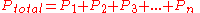,

OR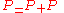,
Where PTotal is the total pressure of the atmosphere,
PGas is the pressure of the gas mixture in the atmosphere,
and PH2O is the water pressure at that temperature.
• Henry's law
Henry's law
In physics, Henry's law is one of the gas laws formulated by William Henry in 1803. It states that:An equivalent way of stating the law is that the solubility of a gas in a liquid at a particular temperature is proportional to the pressure of that gas above the liquid...

states that:
At a constant temperature, the amount of a given gas dissolved in a given type and volume of liquid is directly proportional to the partial pressure
Partial pressure
In a mixture of ideal gases, each gas has a partial pressure which is the pressure which the gas would have if it alone occupied the volume. The total pressure of a gas mixture is the sum of the partial pressures of each individual gas in the mixture....

of that gas in equilibrium with that liquid.